# How to Sum by Formula if Cell Ends with in Excel

We have introduced the method of sum numbers based on criteria “sum if cell begins with” (you can refer to ‘How to Sum Data if Begins with in Excel’ on our website). In this article, we will show you the formula about ‘sum if cell ends with’ with the help of Excel SUMIFS or SUMIF function.

Through demonstrate a simple instance, we will introduce you the syntax, arguments and usage of SUMIFS and SUMIF function, and let you know how this formula works to reach your goal. After reading the article, you may have a simple understanding of SUMIFS/SUMIF function.

## EXAMPLE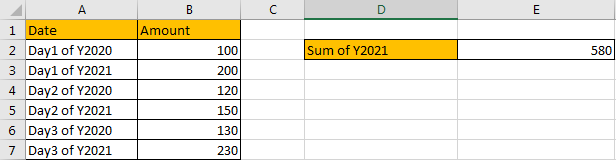Refer to above left-hand table, we can see that there are two columns, one is “Date”, another column is “Amount”. “Date” column lists some dates in year 2020 and 2021. “Amount” column lists the amount for “Date” accordingly.

In this instance, we want to calculate sum of year 2021. So, we need to find out all dates belong to year 2021, and then sum up values in “Amount” column. To find out dates belong to year 2021, through above table we can know that they are ended with 2021, so the filter criteria for “Date” range is “ends with 2021”, after all, we can apply SUMIFS or SUMIF here to fix this issue properly. At first, let’s introduce the usage of SUMIFS formula.

## FORMULA 1 – APPLY SUMIFS FUNCTION

Step 1: Select A2:A7, then in Name Box define a new name for this range, for example ‘Date’.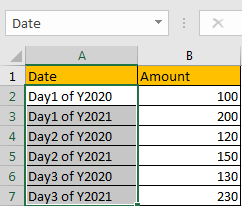Step 2: Select B2:B7, in Name Box define a new name for this range, for example ‘Amount’.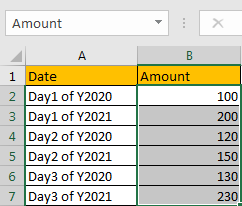Note: Above two steps are optional, you can directly use cell or range reference like A2:A7, B2:B7 in your formula, but for understanding well, we name ranges in advance. When applying formula, user can directly enter “Amount”, “Date” into the formula.

Step 3: In E2, enter the formula =SUMIFS(Amount,Date,”*2021″).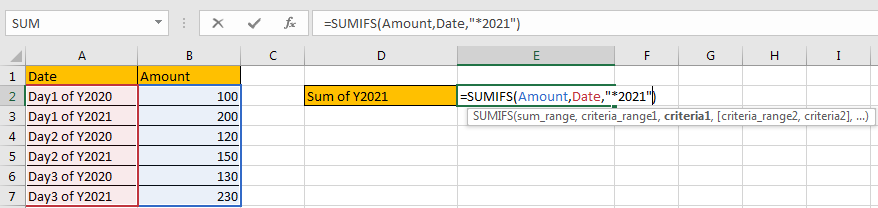Step 4: Press Enter after typing the formula.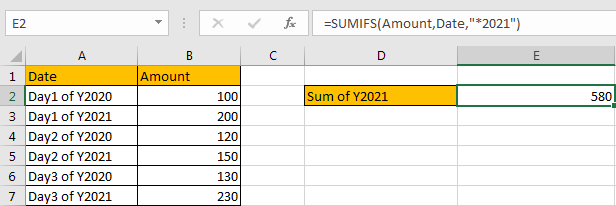We can see in “Date” column, dates in cell A3, A5 and A7 are ended with Y2021, the corresponding amounts are 200 (in cell B3), 150 (in cell B5), and 230 (in cell B7), so the total is 200+150+230=580. The formula works correctly.

## SUMIFS FUNCTION INTRODUCTION

SUMIFS function can be seen as SUM+IFS, it can handle multiple ‘criteria range’ and ‘criteria’ combinations.

For SUMIFS function, the syntax is:

SUMIFS(sum_range, criteria_range1, criteria1, [criteria_range2, criteria2], …). Contents in [] are optional.

SUMIFS function supports wildcards like asterisk ‘*’ and question mark ‘?’, it also supports logical operators within its arguments. If wildcards or logical operators are required, they should be enclosed into double quotes (““) with text.

The usage of wildcards:

1. An asterisk (*) means one or more characters.
2. A question mark (?) means one character.
3. The position of asterisk or question mark means the character(s) position relative to the entered part.

The usage of logical operators:

1. “>” – greater than
2. “<” – less than
3. “<>” – not equal to

### SUMIFS ARGUMENTS EXPLANATION

SUMIFS – SUM RANGE

In our instance, B2:B7 is the ‘sum range’ obviously. Amount values are listed in this field. We define this range with name ‘Amount’ in above step#2.

In the formula bar, select ‘Amount’, press F9, values in this range are listed in an array.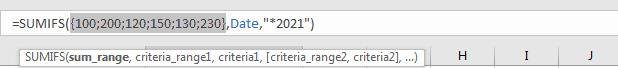SUMIFS – CRITERIA RANGE 1

A2:A7 is the criteria range. In this instance we have only one criteria range. This range contains different dates in year 2020 and year 2021.

In the formula bar, select ‘Date’, press F9. All dates are displayed in proper order in an array: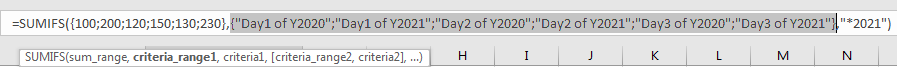SUMIFS – CRITERIA 1

We want to calculate “sum of year 2021”, so we need to find out all dates that ends with year 2021. SUMIFS function supports texts and wildcards, they should be enclosed into double quotes “ “ when applying them, so for criteria 1, we enter “*2021”, “*” means there are some characters before “2021”.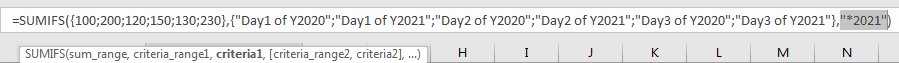SUMIFS WORKFLOW

After explaining each argument in the formula, now we will show you how the formula works with these arguments.

Refer to above mentioned arguments, we can get below formula:

`=SUMIFS({100;200;120;150;130;230},{"Day1 of Y2020";"Day1 of Y2021";"Day2 of Y2020";"Day2 of Y2021";"Day3 of Y2020";"Day3 of Y2021"},"*2021")`

There is one pair of criteria range and criteria:

Criteria Range 1: {“Day1 of Y2020″;”Day1 of Y2021″;”Day2 of Y2020″;”Day2 of Y2021″;”Day3 of Y2020″;”Day3 of Y2021”}

Criteria 1: “*2021”

In criteria range 1, compare each value in the array with criterion “*2021”; if date is ended with 2021, mark them in bold:

{“Day1 of Y2020″;”Day1 of Y2021“;”Day2 of Y2020″;”Day2 of Y2021“;”Day3 of Y2020″;”Day3 of Y2021“}

So, for dates which matches the criteria, record a ‘True’ in the array; otherwise, record a ‘False’. Then we can get a new array:

{False;True;False;True;False;True}

Convert ‘True’ to ‘1’ and ‘False’ to ‘0’:

{0;1;0;1;0;1}

Now, we have below two arrays:

Sum range: {100;200;120;150;130;230}

Criteria: {0;1;0;1;0;1} – from above steps we know that if date ends with “2021”, 1 is displayed.

We list the two arrays in two rows, and multiply the two numbers in the same column, and save their product in “Product” row, then sum all values in “Product”.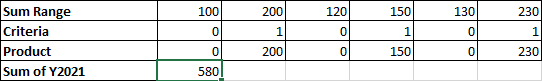Refer to above table, we finally get 580 as returned value.

In this case, asterisk (*) represents one or more characters, if “*” is omit in criteria, that means cell which is exactly equal to “2021” can satisfy the condition. So, if we enter =SUMIFS(Amount,Date,”2021″) to sum numbers, it doesn’t work.

## FORMULA 2 – APPLY SUMIF FUNCTION

Now, let’s try to apply SUMIF function to resolve this issue. The first two steps are the same.

Step 3: In E3, enter the formula =SUMIF(Date,”*2021″,Amount).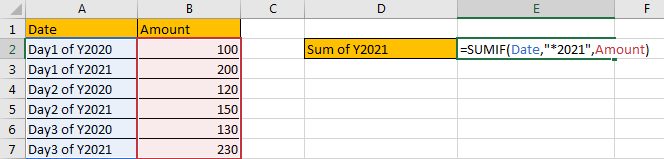Note: We can see in SUMIF formula, we use the same parameters compare with SUMIFS, the difference is range and criteria are moved to the first two positions.

Step 4: Press Enter after typing the formula. Verify that we get the same result.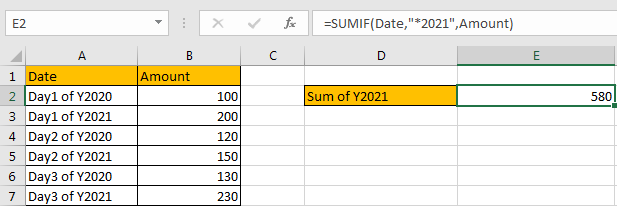## SUMIF FUNCTION INTRODUCTION

SUMIF function can be seen as SUM+IF, it sums numbers based on criteria. it allows user provides one pair of ‘criteria range’ and ‘criteria’.

For SUMIF function, the syntax is:

SUMIF(range, criteria, [sum_range]) – if sum range=range, then sum range can be omitted.

SUMIF function allows wildcards like asterisk ‘*’ and question mark ‘?’, it also allows logical operators within its argument. If wildcards or logical operators are required, they should be enclosed into double quotes (““).

### SUMIF ARGUMENTS EXPLAINATION

SUMIFS and SUMIF share the same parameters in this instance.

SUMIF – RANGE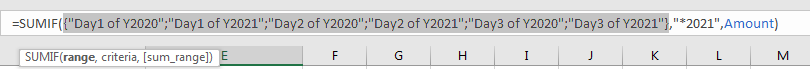SUMIF – CRITERIA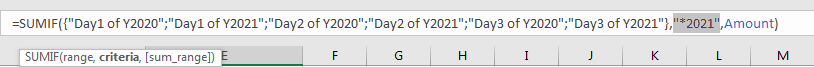SUMIF – SUM RANGE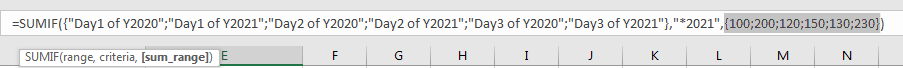SUMIF WORKFLOW

SUMIFS and SUMIF have the same criteria range, criteria and sum range, they are processed with the same steps.

SUMMARY

1. SUMIFS function can handle multiple pairs of criteria ranges and criteria. Sum range is the first argument among all arguments.

2. SUMIF function can handle one pair of criteria range and criteria. Sum range is the last argument among all arguments.

3. They allow user defined range name, wildcards, logical operators.

### Related Functions

• Excel SUMIFS Function
The Excel SUMIFS function sum the numbers in the range of cells that meet a single or multiple criteria that you specify. The syntax of the SUMIFS function is as below:=SUMIFS (sum_range, criteria_range1, criteria1, [criteria_range2, criteria2], …)…
• Excel SUMIF Function
The Excel SUMIF function sum the numbers in the range of cells that meet a single criteria that you specify. The syntax of the SUMIF function is as below:=SUMIF (range, criteria, [sum_range])…